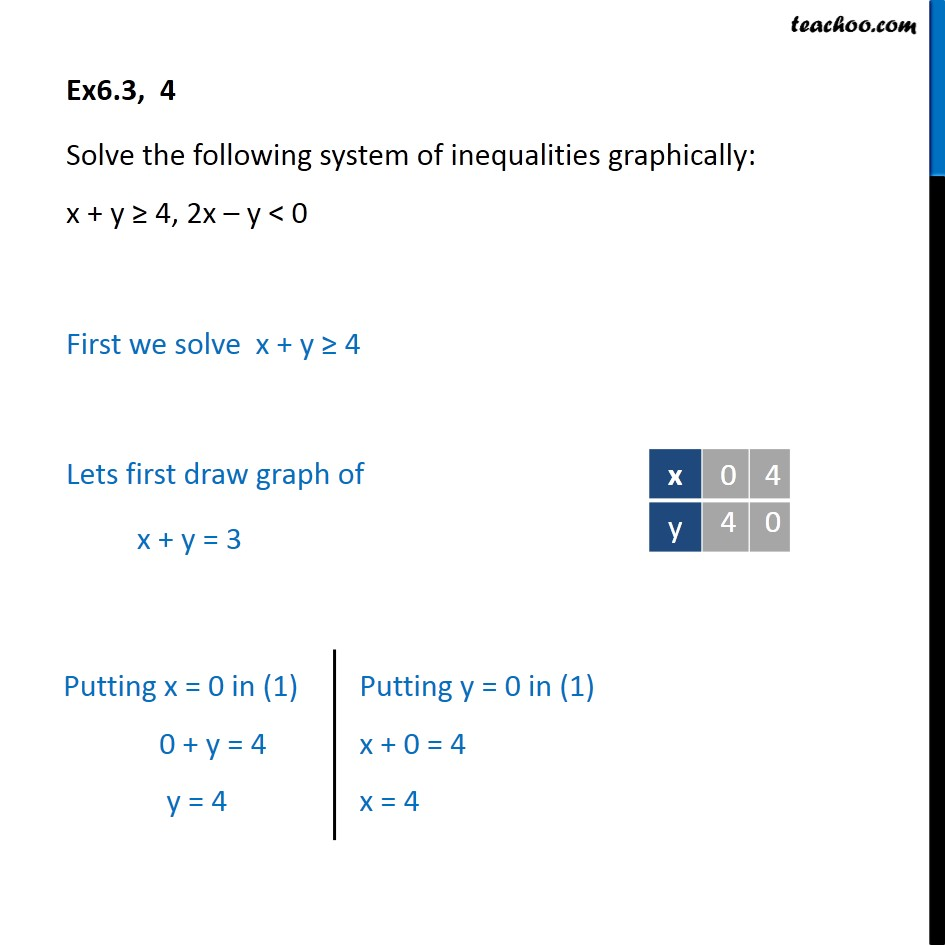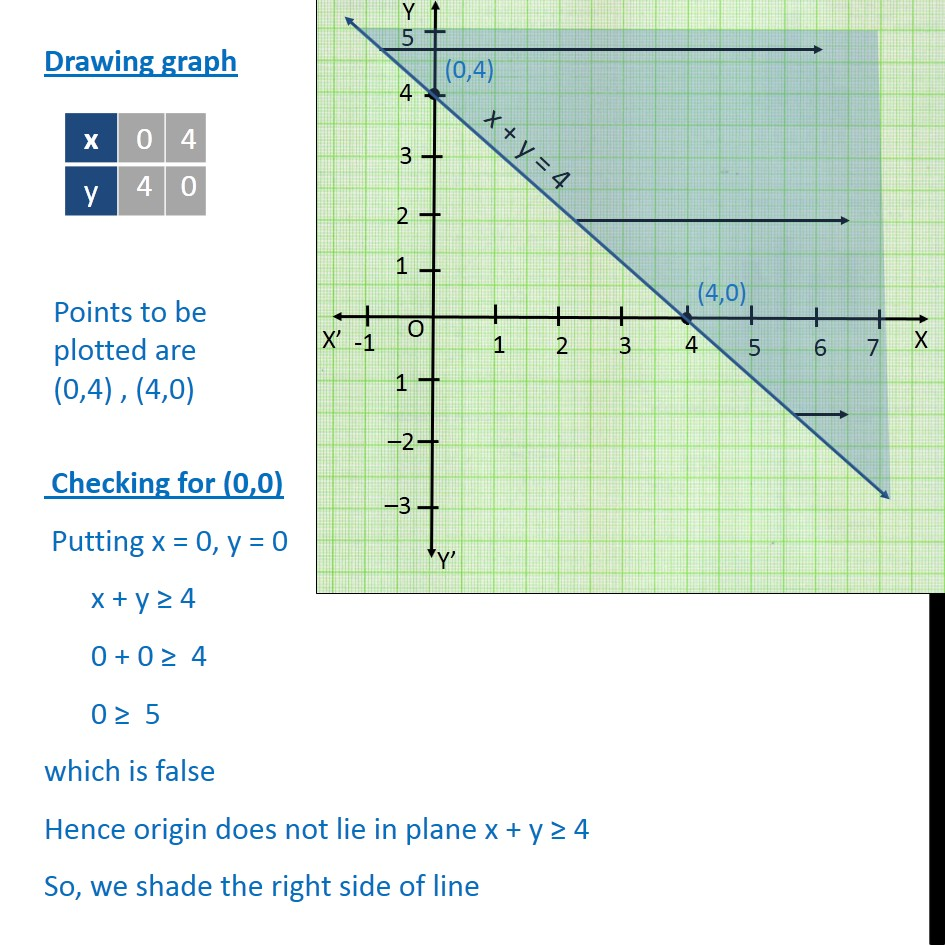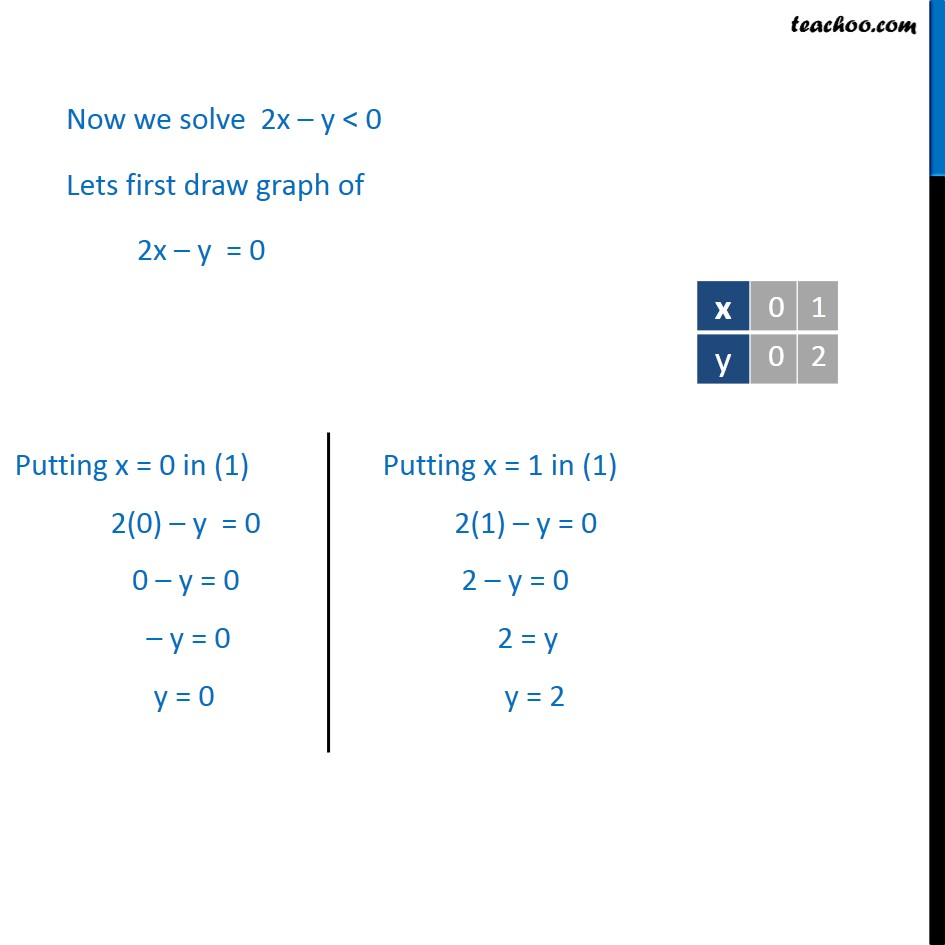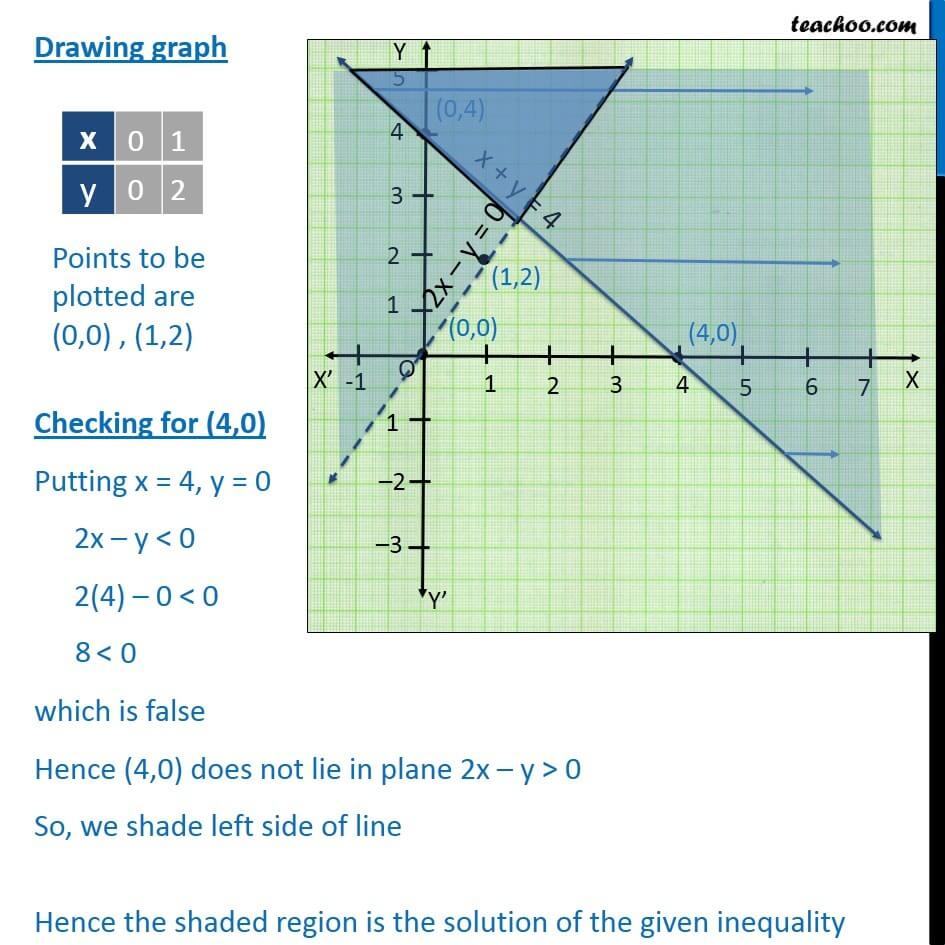Solving Pair of Linear Inequalities

Chapter 5 Class 11 Linear Inequalities
Serial order wiseLearn in your speed, with individual attention - Teachoo Maths 1-on-1 Class

### Transcript

Ex6.3, 4 Solve the following system of inequalities graphically: x + y ≥ 4, 2x – y < 0 First we solve x + y ≥ 4 Lets first draw graph of x + y = 3 Drawing graph Checking for (0,0) Putting x = 0, y = 0 x + y ≥ 4 0 + 0 ≥ 4 0 ≥ 5 which is false Hence origin does not lie in plane x + y ≥ 4 So, we shade the right side of line Now we solve 2x – y < 0 Lets first draw graph of 2x – y = 0 Drawing graph Checking for (4,0) Putting x = 4, y = 0 2x – y < 0 2(4) – 0 < 0 8 > 0 which is false Hence (4,0) does not lie in plane 2x – y > 0 So, we shade left side of line Hence the shaded region is the solution of the given inequality